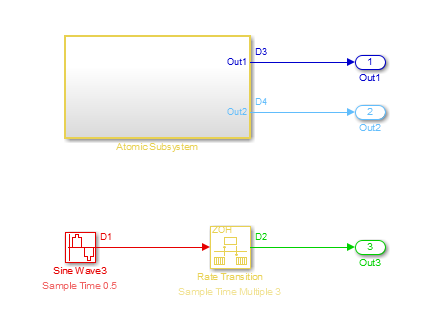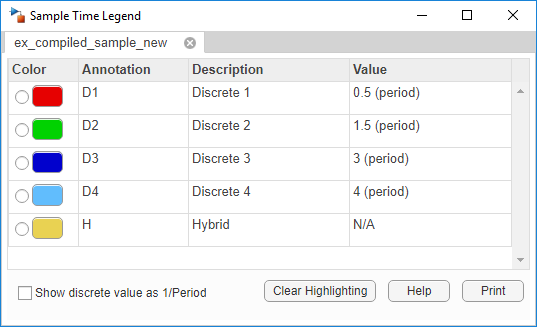## Block Compiled Sample Time

During the compilation phase of a simulation, Simulink® determines the sample time of a block from the `SampleTime` parameter (if the block has an explicit sample time), the block type (if it has an implicit sample time), or by the model content. This compiled sample time determines the sample rate of a block during simulation. You can determine the compiled sample time of any block in a model by first updating the model and then getting the block `CompiledSampleTime` parameter, using the `get_param` command.

For example, consider the model `ex_compiled_sample_new`.Use `get_param` to obtain the block `CompiledSampleTime` parameter for each of the blocks in this example.

`get_param('model_name/block_name','CompiledSampleTime');`
For the Sine Wave3 block,
`get_param('ex_compiled_sample_new/Sine Wave3','CompiledSampleTime');`
displays
`0.5000 0`
The atomic subsystem contains sine wave blocks with sample times of 3 and 4.When calculating the block `CompiledSampleTime` for this subsystem, Simulink returns a cell array of the sample times present in the subsystem.

```3 0 4 0```
The greatest common divisor (GCD) of the two rates is 1. However, this is not necessarily one of the rates in the model.

The Rate Transition block in this model serves as a Zero-Order Hold. Since the `Sample Time Multiple` parameter is set to 3, the input to the rate transition block has a sample rate of 0.5 while the output has a rate of 1.5.

```rt=get_param('ex_compiled_sample_new/Rate Transition',... 'CompiledSampleTime'); rt{:} 0.5000 0 1.5000 0```

The Sample Time Legend shows all of the sample rates present in the model.To inspect compiled sample times throughout a model, you can use the Model Data Editor (on the Modeling tab, click Model Data Editor). After you update the block diagram, the right side of the Sample Time column shows compiled sample times for signals and data stores. For more information, see Model Data Editor.